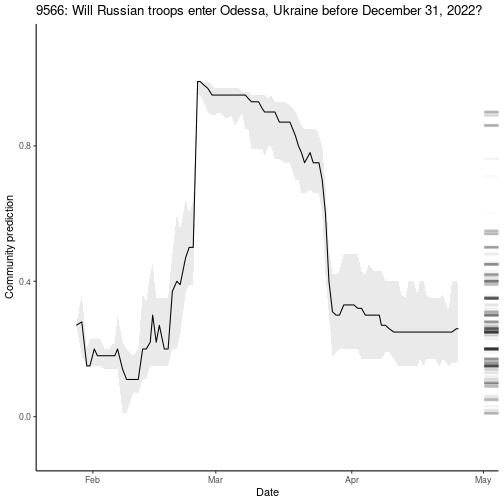# MetaculR

library(MetaculR)

questions_myPredictions <- MetaculR_myPredictions(guessed_by = Metaculus_response_login$Metaculus_user_id) # Analyze Your Predictions ## How different is my last prediction from current community? questions_myPredictions_byDiff <- MetaculR_myDiff(questions_myPredictions) questions_myPredictions_byDiff %>% dplyr::select(id, title, my_prediction, community_q2, community_q2_pre_me, diff_me_q2_abs_odds) %>% dplyr::mutate(diff_me_q2_abs_odds = round(diff_me_q2_abs_odds, 1)) %>% dplyr::arrange(dplyr::desc(diff_me_q2_abs_odds)) %>% head() %>% knitr::kable() id title my_prediction community_q2 community_q2_pre_me diff_me_q2_abs_odds 1634 Will US Income Inequality Increase by 2025? 0.01 0.51 0.53 103.0 1332 Will global malaria mortality rates be reduced by 90% when compared with 2015 rates, by 2030? 0.01 0.44 0.46 77.8 10574 Will a 8-year gross world product doubling complete before a 2-year doubling does? 0.99 0.66 0.75 51.0 8334 Will spending on US office construction be less than$77 Billion USD in 2022? 0.01 0.30 0.30 42.4
10084 Will Sweden join NATO before 2024? 0.14 0.87 0.42 41.1
10085 Will Finland join NATO before 2024? 0.26 0.90 0.83 25.6

### Plot those differences

questions_myPredictions_byDiff %>%
dplyr::arrange(dplyr::desc(diff_me_q2_abs_odds)) %>%
dplyr::slice_head(n = 10) %>%
ggplot2::ggplot() +
ggplot2::geom_col(
ggplot2::aes(x = reorder(factor(id), -diff_me_q2_abs_odds),
y = diff_me_q2_abs_odds,
fill = reorder(factor(id), -diff_me_q2_abs_odds))
) +
ggplot2::theme_classic() +
ggplot2::labs(x = "ID",
y = "Odds difference between me and community q2") +
ggplot2::guides(fill = FALSE) +
ggplot2::theme(axis.text.x = ggplot2::element_text(angle = 45, vjust = 1, hjust = 1))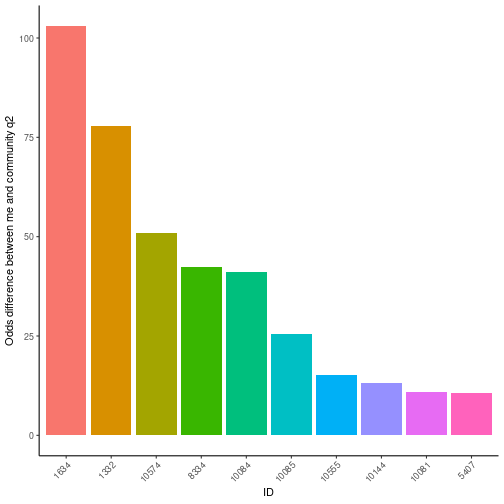plot of chunk unnamed-chunk-8

## Where has community moved most since my last prediction?

questions_myPredictions_byDiff %>%
dplyr::select(id, title, my_prediction, community_q2, community_q2_pre_me, diff_comm_q2_pre_me_abs_odds) %>%
dplyr::mutate(diff_comm_q2_pre_me_abs_odds = round(diff_comm_q2_pre_me_abs_odds, 1)) %>%
dplyr::arrange(dplyr::desc(diff_comm_q2_pre_me_abs_odds)) %>%
knitr::kable()
id title my_prediction community_q2 community_q2_pre_me diff_comm_q2_pre_me_abs_odds
10084 Will Sweden join NATO before 2024? 0.14 0.87 0.42 9.2
9994 Will at least 5 million refugees from Ukraine seek assistance from other countries? 0.99 0.99 0.94 6.3
10057 Will Russia control Chernihiv on June 1, 2022? 0.01 0.01 0.05 5.2
10667 Will Elon Musk hold major political office in the United States before February 2033? 0.01 0.04 0.16 4.6
10004 Will a major nuclear power plant in Germany be operational on June 1, 2023? 0.10 0.25 0.55 3.7
10492 Will Mariupol be under Russian control on June 1, 2022? 0.99 0.95 0.85 3.4

### Plot those differences

MetaculR_plot(MetaculR_questions = questions_myPredictions,
Metaculus_id = 9939)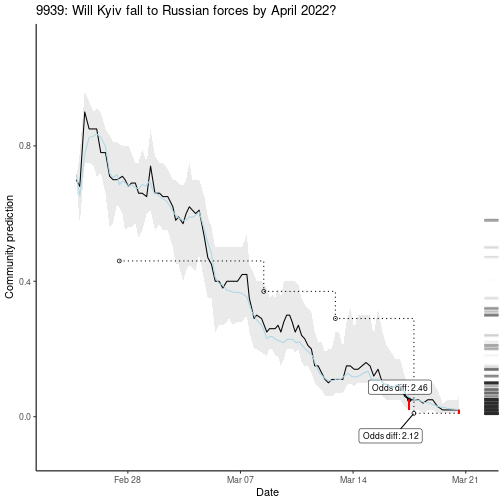plot of chunk unnamed-chunk-10

MetaculR_plot(MetaculR_questions = questions_myPredictions,
Metaculus_id = 6604,
scale_binary = "logodds")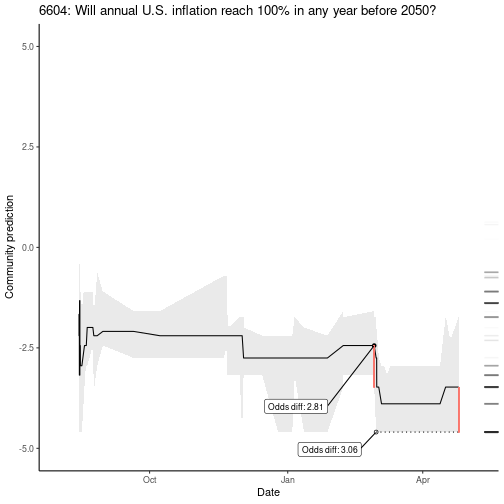plot of chunk unnamed-chunk-11

## Score Predictions

Let’s see some Brier statistics on resolved questions:

# questions_myPredictions_resolved <- MetaculR_myPredictions_Resolved(guessed_by = Metaculus_response_login$Metaculus_user_id) brier_me <- MetaculR_brier(questions_myPredictions) #> Warning in x$results$possibilities$type == "binary" & !is.na(x$results$resolution) & : longer object length is not a multiple
#> of shorter object length

#> Warning in x$results$possibilities$type == "binary" & !is.na(x$results$resolution) & : longer object length is not a multiple #> of shorter object length #> Warning in x$results$possibilities$type == "binary" & !is.na(x$results$resolution) & : longer object length is not a multiple
#> of shorter object length

#> Warning in x$results$possibilities$type == "binary" & !is.na(x$results$resolution) & : longer object length is not a multiple #> of shorter object length #> Warning in x$results$possibilities$type == "binary" & !is.na(x$results$resolution) & : longer object length is not a multiple
#> of shorter object length

#> Warning in x$results$possibilities$type == "binary" & !is.na(x$results$resolution) & : longer object length is not a multiple #> of shorter object length #> Warning in x$results$possibilities$type == "binary" & !is.na(x$results$resolution) & : longer object length is not a multiple
#> of shorter object length

#> Warning in x$results$possibilities$type == "binary" & !is.na(x$results$resolution) & : longer object length is not a multiple #> of shorter object length ### Plot scores brier_me$brier_df %>%
ggplot2::ggplot() +
ggplot2::geom_col(
ggplot2::aes(x = name,
y = value,
fill = ID),
position = "dodge2"
) +
ggplot2::geom_text(
ggplot2::aes(x = name,
y = value,
label = round(value, 3)),
size = 2,
position = ggplot2::position_dodge2(width = 1), #"dodge2",
vjust = 0.5,
hjust = -0.25,
angle = 90) +
ggplot2::theme_classic() +
ggplot2::labs(x = "Statistic",
y = "Value") +
ggplot2::coord_cartesian(ylim = c(-1, 1)) +
ggplot2::theme(axis.text.x = ggplot2::element_text(angle = 45, vjust = 1, hjust = 1))
#> Warning: Removed 17 rows containing missing values (geom_col).
#> Warning: Removed 17 rows containing missing values (geom_text).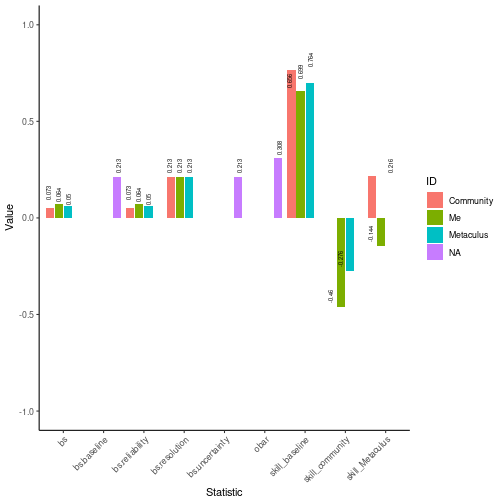plot of chunk unnamed-chunk-14

brier_me$brier_bins_df %>% ggplot2::ggplot() + ggplot2::geom_col( ggplot2::aes(x = centers, y = freqs, fill = ID), position = ggplot2::position_dodge2(width = 0.1, preserve = "single") ) + ggplot2::geom_text( ggplot2::aes(x = centers, y = freqs, label = round(freqs, 3)), size = 2, position = ggplot2::position_dodge2(width = 0.1), #"dodge2", # vjust = 0.5, hjust = -0.25, angle = 90) + ggplot2::theme_classic() + ggplot2::coord_cartesian(ylim = c(0, 1))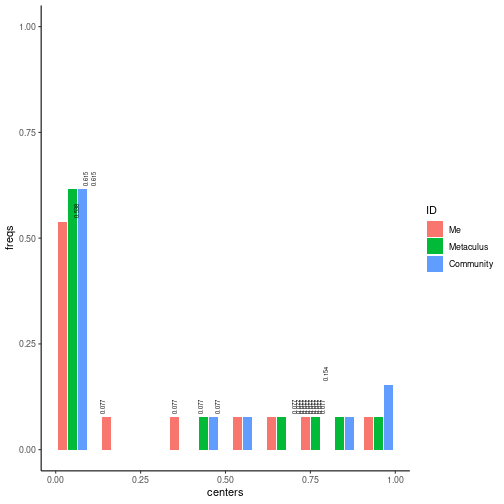plot of chunk unnamed-chunk-15 #### Calibration brier_me$brier_bins_df %>%
ggplot2::ggplot() +
ggplot2::geom_pointrange(
ggplot2::aes(x = centers,
y = obars,
ymin = ci_low,
ymax = ci_high,
color = ID),
position = ggplot2::position_dodge2(width = 0.02)
) +
ggplot2::geom_line(
ggplot2::aes(x = centers,
y = ideal)
) +
ggplot2::geom_text(
ggplot2::aes(x = centers,
y = obars,
label = format(round(obars, 3), nsmall = 3)),
size = 2,
position = ggplot2::position_dodge2(width = 0.1), #"dodge2",
vjust = -0.5,
hjust = -0.25,
angle = 90) +
ggplot2::theme_classic() +
ggplot2::coord_cartesian(ylim = c(0, max(brier_me$brier_bins_df$obars) * 1.1))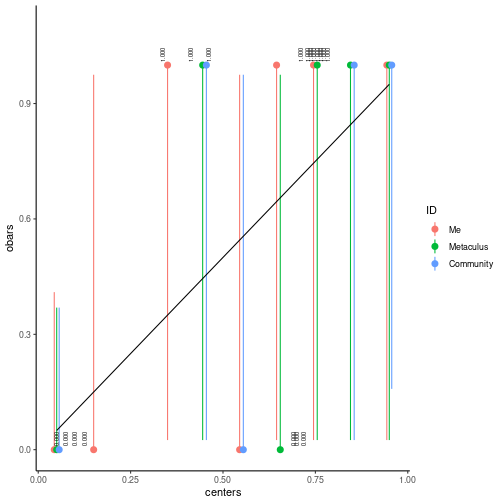plot of chunk unnamed-chunk-16

# Analyze community predictions

For questions you may not have predicted.

## Score, Community Predictions

Let’s see some Brier statistics on resolved questions:

questions_recent_resolved <-
MetaculR_questions(
order_by = "-resolve_time",
status= "resolved",
guessed_by = "",
pages = 32,
offset = 0)
brier_recent_resolved <-
MetaculR_brier(
questions_recent_resolved,
me = FALSE)

### Plot scores, Community Predictions

brier_recent_resolved$brier_df %>% ggplot2::ggplot() + ggplot2::geom_col( ggplot2::aes(x = name, y = value, fill = ID), position = "dodge2" ) + ggplot2::geom_text( ggplot2::aes(x = name, y = value, label = round(value, 3)), size = 2, position = ggplot2::position_dodge2(width = 1), #"dodge2", vjust = 0.5, hjust = -0.25, angle = 90) + ggplot2::theme_classic() + ggplot2::labs(x = "Statistic", y = "Value") + ggplot2::coord_cartesian(ylim = c(-1, 1)) + ggplot2::theme(axis.text.x = ggplot2::element_text(angle = 45, vjust = 1, hjust = 1)) #> Warning: Removed 23 rows containing missing values (geom_col). #> Warning: Removed 23 rows containing missing values (geom_text).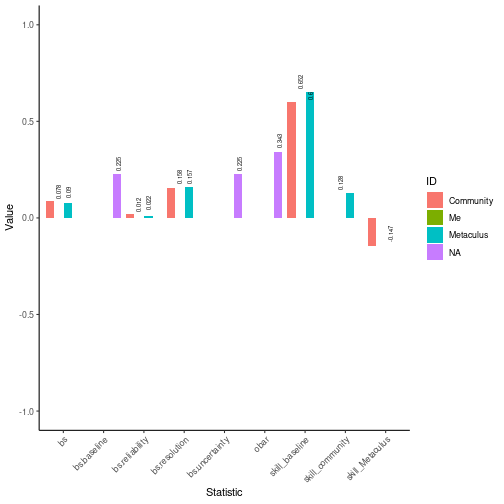plot of chunk unnamed-chunk-19 #### Histogram, Community Predictions brier_recent_resolved$brier_bins_df %>%
ggplot2::ggplot() +
ggplot2::geom_col(
ggplot2::aes(x = centers,
y = freqs,
fill = ID),
position = ggplot2::position_dodge2(width = 0.1)
) +
ggplot2::geom_text(
ggplot2::aes(x = centers,
y = freqs,
label = round(freqs, 3)),
size = 2,
position = ggplot2::position_dodge2(width = 0.1), #"dodge2",
# vjust = 0.5,
hjust = -0.25,
angle = 90) +
ggplot2::theme_classic() +
ggplot2::coord_cartesian(ylim = c(0, max(brier_recent_resolved$brier_bins_df$freqs) * 1.1))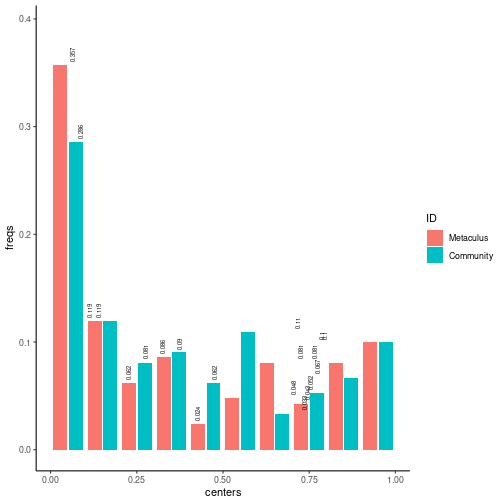plot of chunk unnamed-chunk-20

#### Calibration, Community Predictions

brier_recent_resolved$brier_bins_df %>% ggplot2::ggplot() + ggplot2::geom_pointrange( ggplot2::aes(x = centers, y = obars, ymin = ci_low, ymax = ci_high, color = ID), position = ggplot2::position_dodge2(width = 0.02) ) + ggplot2::geom_line( ggplot2::aes(x = centers, y = ideal) ) + ggplot2::geom_text( ggplot2::aes(x = centers, y = obars, label = format(round(obars, 3), nsmall = 3)), size = 2, position = ggplot2::position_dodge2(width = 0.02), #"dodge2", vjust = -0.5, hjust = -0.25, angle = 90) + ggplot2::theme_classic() + ggplot2::coord_cartesian(ylim = c(0, max(brier_recent_resolved$brier_bins_df\$obars) * 1.1))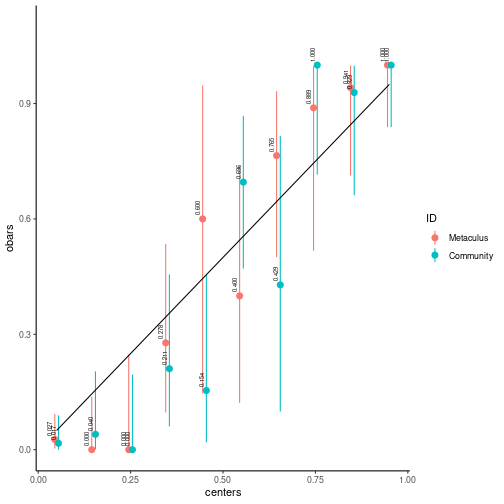plot of chunk unnamed-chunk-21

# Find Exciting Questions

Various sports now have an “Excitement Index” to identify games that interest fans–maybe we can do the same for predictions?

## Among your predictions

questions_myPredictions_byExcitement <- MetaculR_excitement(questions_myPredictions)

questions_myPredictions_byExcitement %>%
dplyr::mutate(Total_logodds_Change = round(Total_logodds_Change, 2),
Total_logodds_Change_Even = round(Total_logodds_Change_Even, 2)) %>%
dplyr::arrange(dplyr::desc(Total_Change)) %>%
knitr::kable()
id title Total_Change Total_logodds_Change Total_Change_Even Total_logodds_Change_Even
10448 Will Russia use chemical weapons in Ukraine in 2022? 2.03 9.17 0.71 3.33
10085 Will Finland join NATO before 2024? 0.71 4.26 0.18 1.13
10057 Will Russia control Chernihiv on June 1, 2022? 0.71 6.53 0.09 0.89
10084 Will Sweden join NATO before 2024? 0.69 3.78 0.22 1.19
9994 Will at least 5 million refugees from Ukraine seek assistance from other countries? 0.47 5.17 0.19 1.38
10246 Will a coup or regime change take place in Russia in 2022 or 2023? 0.46 3.67 0.22 1.75
MetaculR_plot(MetaculR_questions = questions_myPredictions,
Metaculus_id = 9790)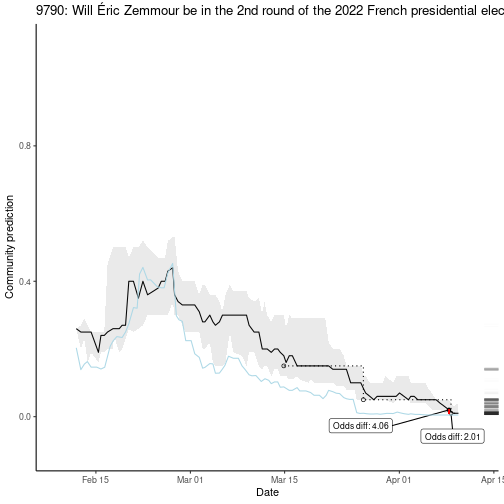plot of chunk unnamed-chunk-23

questions_myPredictions_byExcitement %>%
dplyr::mutate(Total_logodds_Change = round(Total_logodds_Change, 2),
Total_logodds_Change_Even = round(Total_logodds_Change_Even, 2)) %>%
dplyr::arrange(dplyr::desc(Total_logodds_Change)) %>%
knitr::kable()
id title Total_Change Total_logodds_Change Total_Change_Even Total_logodds_Change_Even
10448 Will Russia use chemical weapons in Ukraine in 2022? 2.03 9.17 0.71 3.33
10057 Will Russia control Chernihiv on June 1, 2022? 0.71 6.53 0.09 0.89
9994 Will at least 5 million refugees from Ukraine seek assistance from other countries? 0.47 5.17 0.19 1.38
10094 Will Russia control Kyiv on June 1, 2022? 0.17 4.50 0.03 0.95
10085 Will Finland join NATO before 2024? 0.71 4.26 0.18 1.13
10269 Will Kyiv be under Russian control before 2023? 0.34 4.03 0.04 0.72
MetaculR_plot(MetaculR_questions = questions_myPredictions,
Metaculus_id = 6725)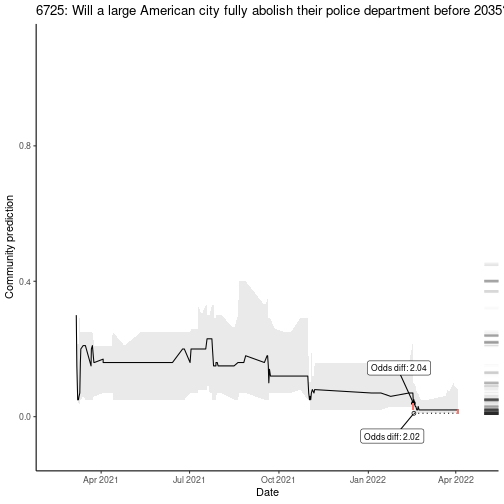plot of chunk unnamed-chunk-25

## Among community predictions

### Resolved questions

What were the most exciting resolved questions?

questions_recent_byExcitement <- MetaculR_excitement(questions_recent_resolved)

questions_recent_byExcitement %>%
dplyr::mutate(Total_logodds_Change = round(Total_logodds_Change, 2),
Total_logodds_Change_Even = round(Total_logodds_Change_Even, 2)) %>%
dplyr::arrange(dplyr::desc(Total_Change_Even)) %>%
knitr::kable()
id title Total_Change Total_logodds_Change Total_Change_Even Total_logodds_Change_Even
6763 Will Marine Le Pen win the 2022 French presidential election? 0.30 3.16 0.16 1.79
10282 Will Russia default on its debt in 2022? 0.46 2.09 0.12 0.49
2511 Will Emmanuel Macron be re-elected President of France in 2022? 0.25 2.24 0.11 0.93
9182 Will more than 20,000 people die within 28 days of a positive Covid test in the UK in 2022? 0.20 1.33 0.03 0.17
9609 Will Viktor Orbán win the 2022 Hungarian Parliamentary Election? 0.17 0.87 0.03 0.15
9790 Will Éric Zemmour be in the 2nd round of the 2022 French presidential election? 0.15 3.50 0.03 0.55
MetaculR_plot(MetaculR_questions = questions_recent_resolved,
Metaculus_id = 8898)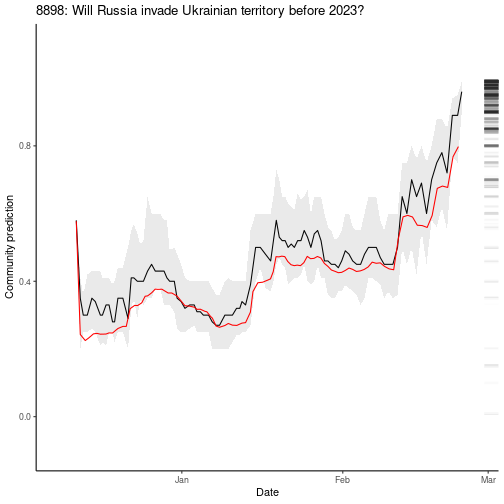plot of chunk unnamed-chunk-27

### Open questions

What are the most exciting questions that are still open?

questions_recent_open <-
MetaculR_questions(
order_by = "close_time",
status = "open",
guessed_by = "")
questions_recent_open_byExcitement <- MetaculR_excitement(questions_recent_open)

questions_recent_open_byExcitement %>%
dplyr::mutate(Total_logodds_Change = round(Total_logodds_Change, 2),
Total_logodds_Change_Even = round(Total_logodds_Change_Even, 2)) %>%
dplyr::arrange(dplyr::desc(Total_logodds_Change_Even)) %>%
knitr::kable()
MetaculR_plot(MetaculR_questions = questions_recent_open,
Metaculus_id = 9566)